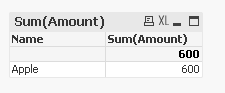# QlikView App Development

Discussion Board for collaboration related to QlikView App Development.

Highlighted
Contributor

## Need Help with IF AND statement

Hi All,

I need some help with an IF AND statement for my chart expressions . I have two
fields where I want to sum the amount if it meets the criteria. To put it in
words, IF Description=”EMV” AND Amount=0, then  Sum everything in the Amount field  Else Description=”EMV” AND Amount >0, Sum
just the SELL field.

See below example.

#1

 Description Amount Buy -1,000 Sell 600 Interest 260 EMV 0 -140

Want to return the sum of amount -140

#2

 Description Amount Buy -1,000 Sell 600 Interest 260 EMV 500 360

Want to return just the Sell for 600

Any inputs will be greatly appreciated.

Thanks,

Frank

Tags (3)
1 Solution

Accepted Solutions
Honored Contributor III

## Re: Need Help with IF AND statement

Try the attached one, it works for both the condition with the sample -

Name, Description,Amount

Apple,Sell,600

Apple,Interest,260

Apple,EMV,200

];10 RepliesPartner

## Re: Need Help with IF AND statement

Hi,

Try below expression:

=Sum(if(match(Desc,'EMV') and Amount<=0,0,Amount))

Hope this helps.

Regards,

Andrei

Contributor

## Re: Need Help with IF AND statement

Hi Andrei,

The AND part didnt works even if i change it to greater or less than zero. It goes to the else and return the Amount.

Let me know if you can help.

Sum(if(match([Description],'EMV') and Amount<=0,0,Amount))Partner

## Re: Need Help with IF AND statement

Hi,

Hm... in which type of chart are you trying to do so? Straight table? Text Object?

What dimensions are there?

Regards,

Andrei

Valued Contributor

## Re: Need Help with IF AND statement

hi,

check this

You can test both scenario by making change in inline table.

Contributor

## Re: Need Help with IF AND statement

Hi Andrei,

It's a straight table with 3 dimensions. See below

 Name Description Amount Apple Buy -1,000 Apple Sell 600 Apple EMV 500 or 0 Apple Interest 260

*** Example EMV - either 500 or 0

Sum(if(match([Description],'EMV') and Amount<=0,0,Amount))

I want to return the amount field with these 2 criterias.

If  Descritpion= EMV and Amount = 0 then
Sum(Amount)

Elseif Descritpion= EMV and Amount > 0 then

Sum(Amount) - Only the SELL's (600)

End if

Hope i didnt confuse you. Many Thanks,
Frank

Contributor

## Re: Need Help with IF AND statement

Hi Ankit,

I did test it out, only works for one scenario. Only returns the Amount even if i change the EMV =0.  Below if statement is what i want to accomplish (also, please see my recent post to Andrei).

If  Descritpion= EMV and Amount = 0 then
Sum(Amount)

Elseif Descritpion= EMV and Amount > 0 then

Sum(Amount) - Only the SELL's (600)

End if

Sum(if(match([Description],'EMV') and Amount=0,0,Amount))

Your formula works if EMV >0  and return sum of Amount. Once i change the EMV value to =0 , still return sum of the Amount.

Many Thanks

FrankPartner

## Re: Need Help with IF AND statement

Hi Frank,

The second condition seems a bit confusing, but have a look on my expression:

if(match(Description,'EMV') and Amount=0, sum(TOTAL Amount), //Means sum(Amount) of all cells(rows)

if( match(Description,'EMV') and Amount>0,

sum(TOTAL Amount)-sum({<Description={'Sell'}>} TOTAL Amount) //Means sum(Amount) of all rows - (minus) Only the SELL's row.. If you need SELL's row only just drop first part before (including) minus

,  sum(Amount)

)

)

Hope this helps or just shed light on the solution)

Regards,

Andrei

Contributor

## Re: Need Help with IF AND statement

Andrei,  Thanks for your help!. I tweeted your formula a bit and still didnt come to a solution that i wanted. Let me know if you can take a look at my sample QVW.

I have a Straight Table  where i use Name, Description and an expression with If statement.  The formula works in a way when i click "Table" Name such as Apple or Orange.  For instance, if i click Apple it returns 360 and Orange returns 700 (only Sell) which both returns are correct with this formula. But when i clear it,  it shows a different numbers.

Also, how can i show Name level in straight table? I tried without the description, no data at all.

for example -

Name  Amount

Apple 360

Orange 700

Many Thanks.

Frank

Honored Contributor III

## Re: Need Help with IF AND statement

Try the attached one, it works for both the condition with the sample -

Name, Description,Amount